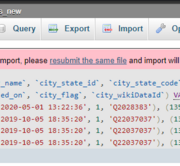# How to Get the First 5 Elements of Array in PHP?

Here, I will show you the php array to get the first 5 elements. let’s discuss about php array getting 5 first element values. you can see how to get 5 first element of the array in PHP. This post will give you a simple example of PHP getting 5 first key of an array example.

we will use the array_slice() function to get the first 5 elements of an array in PHP. so, let’s see the simple code of how to get the first 5 values in a php array.

## Example:

``````<?php

\$myArray = ["One", "Two", "Three", "Four", "Five", "Six", "Seven", "Eight"];

\$firstElems = array_slice(\$myArray, 0, 5);

var_dump(\$firstElems);

?>``````

## PHP explode() Function Example Tutorial

A PHP explode function example is given in this article. I will demonstrate how to use PHP’s explode string to array function. This is a basic example…

## How to Convert Array to String in PHP?

We will cover the example of converting an array to a string in PHP in this lesson. We’ll examine an example of converting an array to a…

## How Can I Convert a String in PHP Into an Array?

We will go over how to transform a string into an array in PHP in this little tutorial. Describe how to convert a string value into an…

## How to Use the array_merge() Function in PHP?

We will go over the simple and quick method to use the PHP array_merge() function example in this brief tutorial. Let’s talk about the PHP example of…

## How to Merge Two Arrays in PHP?

I explained simply how to merge two arrays in PHP Laravel. you will learn how to append two arrays in PHP. you will learn how to merge…In this article we have shown How to fix the “Script timeout passed, if you want to finish import, please resubmit the same file and import will…